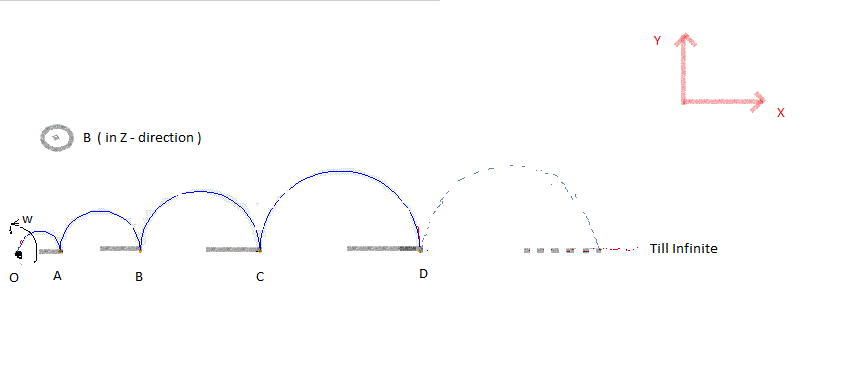# Magnetism + Sequence = MaquenceAn infinitely long, thin, conducting rod is bent in the form of semi-circular rings, as shown in the figure. Now it is placed in a uniform magnetic field of magnitude $B_0$ oriented perpendicular to the plane of the rings.

The radii of the successive half rings are as follows: $R, 3R^2,5R^3,7R^4 \ldots \infty$

Now if we rotate the Rod about the point $O$ with constant angular velocity $\omega$, find the magnitude of the induced emf developed in the rod.

Assumptions

• The rod is very thin.
• The rod is rigid.

Details

• $B _0=2.0$ Tesla
• $\omega =5$ rad/sec
• $R=0.5$ m

×